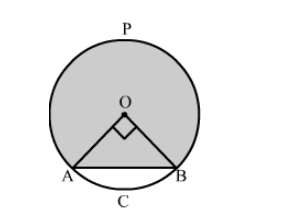# A chord of a circle of radius 10 cm subtends a right angle at the centre.`
Question:

A chord of a circle of radius 10 cm subtends a right angle at the centre. The area of the minor segments (given, π = 3.14) is
(a) 32.5 cm2
(b) 34.5 cm2
(c) 28.5 cm2
(d) 30.5 cm2

Solution:Area of minor segment = Area of sector AOBC − Area of right triangle AOB

$=\frac{90^{\circ}}{360^{\circ}} \pi(\mathrm{OA})^{2}-\frac{1}{2} \times \mathrm{OA} \times \mathrm{OB}$

$=\frac{1}{4} \times 3.14 \times(10)^{2}-\frac{1}{2} \times 10 \times 10$

$=78.5-50$

$=28.5 \mathrm{~cm}^{2}$

Hence, the correct answer is option (c).